# All Posts

In Graph Convolutional Networks and Explanations, I have introduced our neural network model, its applications, the challenge of its “black box” nature, the tools we can use to better understand it, and the datasets we can use to validate those tools. The two tools mentioned are feature visualization and attribution. Both are rich topics and each deserves its own blog post.

Feature Visualization

We have a trained neural network model and we want to understand what it does. One idea is to try figuring out what each neuron represents. We know that for the output layer the neurons represent each of the class labels. But the semantics of the internal neurons are left up to the model to define. We can get a pretty good understanding, however, by looking for the input that maximizes the activation of the given neuron.

Feature Visualization: How neural networks build up their understanding of images, by Chris Olah, Alexander Mordvintsev, and Ludwig Schubert is a beautifully illustrated article on applying this approach to image classifier neural networks. An example from their work is an input that maximizes the activation of a neuron associated with dogs: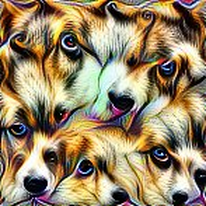Such illustrations helps us understand what features the network relies on to identify a given class. We can deduce that the eyes, noses, and furry textures are important, while symmetry is not a concern. As this example suggests, feature visualization yields insightful results even when used on neurons of the final layer.

Feature visualization starts with a random input and adjusts it along the gradients propagated back from the target neuron. Put very concisely, instead of training the model parameters to give the desired answer, we train the input image.

We applied the same idea to GCNs.

Our random input is a 10-vertex complete graph with randomized features. We adjust the edge weights and vertex features according to the gradients until we arrive at an input that highly activates the neuron representing the class we are interested in.

It worked great on the “two steps from a rockstar” dataset.

Two examples: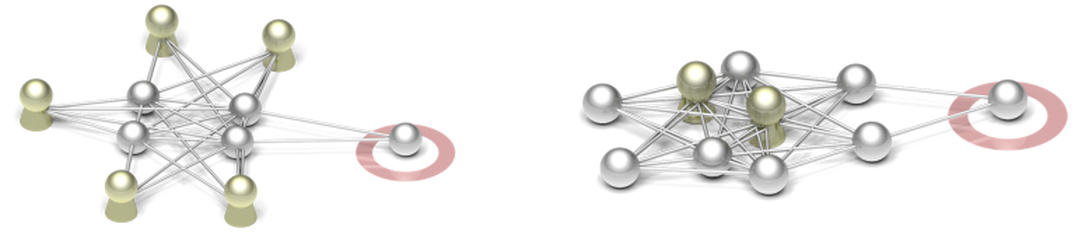In both cases we optimized the input to give the vertex in the red circle the positive label. Recall from Graph Convolutional Networks and Explanations, that the rule in this dataset was that the vertex would have to be exactly two steps away from a rockstar. We can see that feature visualization was able to generate examples that clearly demonstrate this rule.

These pictures are proof that the GCN was able to learn this rule, and that feature visualization was able to effectively extract this rule from the model in the form of a number of examples.

It performed less perfectly on the “three rockstar friends” dataset: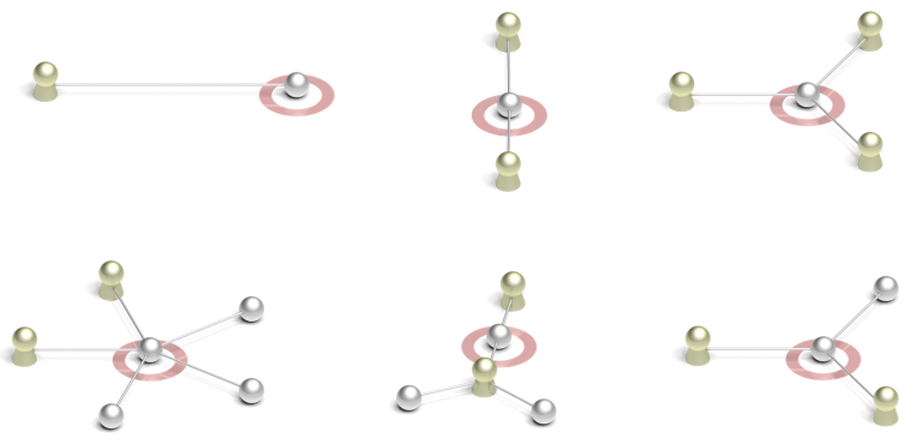In most cases the generated example only has one or two rockstar neighbors. The rule was to have three rockstars. What went wrong?

It is easy to confirm that the model gives a high-confidence positive classification for these examples. The issue is with the model, not with feature visualization.

The training dataset is based on a real-world social network structure. Most vertices have 50-100 neighbors. Our current model architecture uses the symmetric normalization approach from Kipf & Welling (ICLR 2017), so it cannot make accurate counts of neighbors. The model can only learn that some percentage of neighbors must to be rockstars. When we generate an example with only 10 vertices, this often results in less than three rockstar neighbors.

Feature visualization in this case has not only explained to us how the model makes its decisions, but it has also highlighted a shortcoming of the model. It would not transfer well to datasets with a different degree distribution.

Once we were confident in feature visualization’s ability to recover rules from the model, we tried it on a real-world dataset: age prediction. Here people are placed into four age buckets (10–20, 20–24, 24–29, 29–69), each containing 25% of the population. The age data is discarded for a part of the population and the task is to give predictions for these people.

The first feature visualizations were disappointing: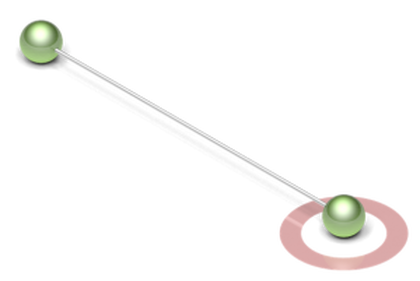This is an example input that maximizes the confidence of belonging to the 24–29 age bucket. It has a single neighbor that belongs to that bucket. Of course this is a good example. It’s a person that has 100% of their neighbors in the given age bucket. 99.2% confidence.

An example for the 29–69 age bucket: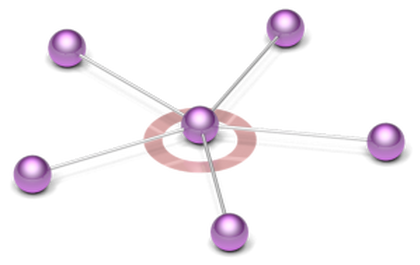99.9998% confidence! The difference is due to the symmetric normalization being a bit more complicated than just taking the average of neighbor’s values, and this being a different age bucket.

The problem is, these examples are so obvious!

These examples suggest that the model just predicts whichever age bucket is most common among the vertex’s neighbors. We actually use that approach as one of the baseline human-crafted models. And the GCN always outperforms this model. So there must be more to its strategy. But it may still use this simple strategy in a large number of cases.

Thankfully, feature visualization outputs an endless number of different examples due to the random initialization. After looking through dozens of them, I found a number of more interesting cases. I took a closer look at one interesting example: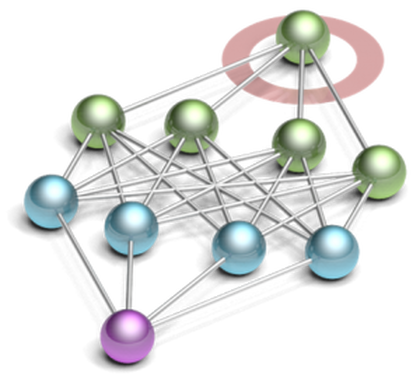In line with the previous examples, here we have an example optimized to be classified into the 24–29 age bucket that has all of its neighbors in the same age bucket. But beyond those immediate neighbors we have another layer of second-degree neighbors from the 10–20 age bucket, and a vertex from the 29–69 bucket three steps away.

Are the vertices two and three steps away just noise? Or do they actually contribute to classifying the targeted vertex into the targeted age bucket?

To find out, I simply took away the vertices one by one and calculated the confidence of the classification at each step.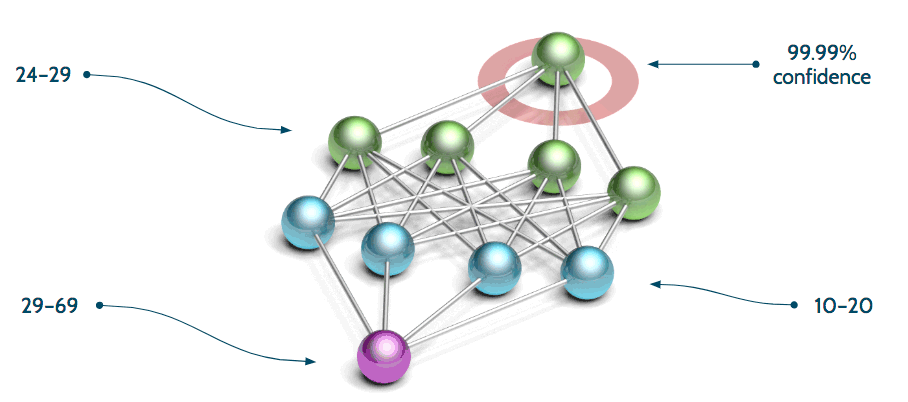There is a gradual decrease from 99.99% to 99.93% as I remove the vertices in the indirect neighborhood. This means there is a weak, but real effect from them. The model predicts higher probability for belonging into the 24–29 age bucket when the indirect neighbors are present.

Feature visualization has helped us discover something exciting and real about the model. And the model was trained on real data. It must have seen a pattern like this in the real data, and feature visualization has revealed this to us.

We plan on pursuing a number of feature visualization applications as a continuation of this research:

• We want to try extracting rules from the data in a practical use case. Such rules can then inform decision-making.

• We intend to use it for finding the weak points of our models. (Such as in the “three rockstar friends” case above.)

• It is helpful for research on model architectures. We have interrogated neurons in hidden layers and found it useful for testing theories about the model’s behavior.

• It is helpful for research on the training process. Seeing a sharp drop or spike in the training loss curve we used to wonder what happened there. With feature visualization, we can check examples before and after the change and understand what happened.

• The same “backpropagation on the input” trick (and same code) can be used starting from real data instead of starting from a small randomized state. The algorithm would then tell us how the vertex neighborhood would have to change to place the vertex in a different class. Not of practical use when the model is predicting the user’s age, but absolutely useful when the model is predicting whether a user will upgrade their subscription!

Feature visualization gives us examples of concepts that the neural network has learned. We can use it to better understand the network in general. But when we have a specific input and the network makes a classification, we can ask more specific questions. Attribution is the question of which parts of the input have led to that classification.

Coming up next: Attribution on a GCN

See All